# Hardest colleges to get into in New York

Top 10 colleges in New York with the lowest acceptance rates
What are the hardest colleges to get into in New York? We've got you covered. We've compiled a national college database and have created a list of the hardest universities to get into in New York below. These are the hardest 4 year colleges to get into in New York, and sometimes some of the hardest colleges to get into in the US. You could even say these are the best colleges in New York. We also include each college's average SAT and ACT scores so that you can see where you're most competitive. Read on to find out more.

## Columbia University in the City of New York acceptance rate

Columbia University in the City of New York acceptance rate is 5.9%.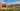The average SAT score for Columbia University in the City of New York is 1505.

• SAT composite: 1505
• SAT math: 770

The average ACT score for Columbia University in the City of New York is 34.

## Cornell University acceptance rate

Cornell University acceptance rate is 10.9%.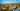The average SAT score for Cornell University is 1480.

• SAT composite: 1480
• SAT math: 760

The average ACT score for Cornell University is 34.

## The Cooper Union acceptance rate

The Cooper Union acceptance rate is 13.1%.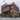Ajay Suresh / Flickr / "The Cooper Union's Foundation Building - North Side" / CC BY 2.0

The average SAT score for The Cooper Union is 1413.

• SAT composite: 1413
• SAT math: 730

The average ACT score for The Cooper Union is 31.

## Barnard College acceptance rate

Barnard College acceptance rate is 13.9%.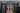The average SAT score for Barnard College is 1404.

• SAT composite: 1404
• SAT math: 700

The average ACT score for Barnard College is 31.

## New York University acceptance rate

New York University acceptance rate is 16.2%.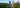The average SAT score for New York University is 1440.

• SAT composite: 1440
• SAT math: 740

The average ACT score for New York University is 32.

## Hamilton College acceptance rate

Hamilton College acceptance rate is 21.3%.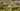The average SAT score for Hamilton College is 1440.

• SAT composite: 1440
• SAT math: 730

The average ACT score for Hamilton College is 33.

## Vassar College acceptance rate

Vassar College acceptance rate is 24.6%.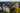The average SAT score for Vassar College is 1440.

• SAT composite: 1440
• SAT math: 730

The average ACT score for Vassar College is 32.

## Colgate University acceptance rate

Colgate University acceptance rate is 24.9%.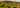The average SAT score for Colgate University is 1415.

• SAT composite: 1415
• SAT math: 725

The average ACT score for Colgate University is 33.

## Skidmore College acceptance rate

Skidmore College acceptance rate is 26.9%.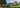The average SAT score for Skidmore College is 1310.

• SAT composite: 1310
• SAT math: 660

The average ACT score for Skidmore College is 29.

## University of Rochester acceptance rate

University of Rochester acceptance rate is 31.4%.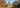The average SAT score for University of Rochester is 1420.

• SAT composite: 1420
• SAT math: 750